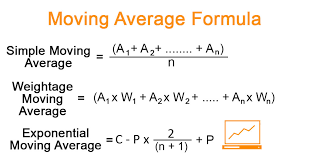# Moving Average - Overview and Its Types

by Ranny Watson BloggerInvestors and market analysts use moving average to determine directions of trends. There are two types of moving average. Each type has a formula to calculate moving average. Market analysts and investors sum up data points over a specific period of time and divide it by the number of data points to calculate moving average. It is recalculated on the basis of the latest data.

The purpose of calculating moving average is the examination of support and resistance by the asset's price movements' evaluation. This evaluation provides information that is used to determine the direction of the price of that asset.

The following are the two types of moving averages:

• Simple Moving Average (SMA)

• Exponential Moving Average (EMA)

## Simple Moving Average (SMA)

In simple moving average, you obtain the recent data points and sum them up. Now, divide the total by the number of data points. It is a simple formula to calculate moving average. Simple moving average is used to determine when to enter a market and when to exit. Simple moving average is considered backward looking. It uses price data over a given period of time in the past. You can compute it for low, high, close and open prices. Investors and analysts in financial markets calculate simple moving average determined signals to buy and sell securities. To generate a simple moving average, you need to select a period of time and add prices over that period. Dividing the sum of prices by the number of periods provides you with SMA. You can use this information to plot a graph.

The following formula is used to calculate simple moving average:

Simple Moving Average (SMA) = (A1 + A2 + A3 + A4 + ……. An)/n

## Exponential Moving Average (EMA)

This type of moving average considers the latest data points. As a result, it is more responsive to the latest changes in price. On the other hand, a simple moving average considers changes in the price over a given period of time.

You can take the following three steps to calculate the exponential moving average:

1. Calculate SMA for the given period.

2. Calculate the multiplier.

3. Calculate the current EMA.

### Step 1: Calculate the SMA

To calculate the moving average of this type, you need a starting point. Investors and analysts use a simple moving average as the previous period's exponential moving average. To obtain this, sum up the closing prices of the security for the given period of time and divide it by the number of periods.

### Step 2: Calculate the Multiplier

Use the following formula to calculate multiplier:

Multiplier = [2/(selected time period+1)]

### Step 3: Calculate the Current EMA

Use the following formula to calculate the current EMA:

Current exponential moving average = [closing price-exponential moving average ( previous time period )] × multiplier + exponential moving average(previous time period)

## Simple Moving Average vs. Exponential Moving Average

Exponential moving average is more sensitive to recent price changes. Therefore, it is more responsive than a simple moving average. The formula for calculating simple moving average is simple and straightforward. On the other hand, the formula for exponential moving average calculation is complicated.

### About Ranny WatsonBlogger

132 connections, 4 recommendations, 485 honor points.
Joined APSense since, April 22nd, 2016, From Boca Raton, FL, United States.Report this Page

Created on Nov 23rd 2020 22:57. Viewed 206 times.Share on APSense